### SineSine, in mathematics, is a trigonometric function of an angle. The sine of an angle is defined in the context of a right triangle: for the specified angle, it is the ratio of the length of the side that is opposite that angle to (divided by) the length of the longest side of the triangle (i.e. the hypotenuse). Trigonometric functions are commonly ...
Found on http://en.wikipedia.org/wiki/Sine

### Sine• (n.) The length of a perpendicular drawn from one extremity of an arc of a circle to the diameter drawn through the other extremity. • (n.) The perpendicular itself. See Sine of angle, below. • (prep.) Without.
Found on http://thinkexist.com/dictionary/meaning/sine/

### sine<mathematics> The length of a perpendicular drawn from one extremity of an arc of a circle to the diameter drawn through the other extremity. ... The perpendicular itself. See Sine of angle, below. Artificial sines, logarithms of the natural sines, or logarithmic sines. Curve of sines. See Sinusoid. Natural sines, the decimals expressing the ...
Found on http://www.encyclo.co.uk/local/20973

### sine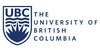(+ abl.) without.
Found on https://www.math.ubc.ca/~cass/frivs/latin/latin-dict-full.html

### sine(from the article `mathematics`) ...variable , then a remarkable new theory became apparent. The new function, for example, possessed a property that generalized the basic property ... Euler`s analytic approach is illustrated by his introduction of the sine and cosine functions. Trigonometry tables had existed since antiquity, and ... ...
Found on http://www.britannica.com/eb/a-z/s/99

### sinesin noun ratio of the opposite side to the hypotenuse of a right-angled triangle
Found on https://www.encyclo.co.uk/local/20974

### sine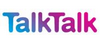Click images to enlargeIn trigonometry, a function of an angle in a right-angled triangle that is defined as the ratio of the length of the side opposite the angle to the length of the hypotenuse (the longest side). This function can be used to find either angles or sides in a right-angled triangle
Found on http://www.talktalk.co.uk/reference/encyclopaedia/hutchinson/m0006736.html

### SineSi'ne preposition [ Latin ] Without.
Found on http://www.encyclo.co.uk/webster/S/106

### SineSine noun [ Late Latin sinus a sine, Latin sinus bosom, used in translating the Arabic jaib , properly, bosom, but probably read by mistake (the consonants being the same) for an original jība sine, from Sanskrit jīva bowstring, chord of an arc, sine.]
Found on http://www.encyclo.co.uk/webster/S/106

### Sinefunction init(){}Note: In this series A is in radians. 7 terms in the series are required to get ananswer accurate to 8 decimal places for . Ten terms in the seriesare required to get an answer accurate to 15 decimal places for the same range of A.There are a number of ways denoting the inverse sine function e.g. sin-1(A) orarcsin(A) or ...
Found on http://www.diracdelta.co.uk/science/source/s/i/sine/source.html

### sineLatin, meaning: (+ abl.) without.
Found on http://archives.nd.edu/sss.htm

### Sine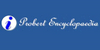Originally sine was another word to describe a gulf or a bay, as in 'The Persian Sine', today its use is more limited to its trigonometry variation which describes the straight line drawn from one extremity of an arc perpendicular to the diameter passing through the other extremity.
Found on http://www.probertencyclopaedia.com/browse/GS.HTM

### sine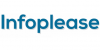sine: see trigonometry.
Found on http://www.infoplease.com/ce6/sci/A0918781.html

### sine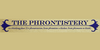without
Found on http://phrontistery.info/s.html

### sine[n] - ratio of the opposite side to the hypotenuse of a right-angled triangle
Found on http://www.webdictionary.co.uk/definition.php?query=sine
No exact match found